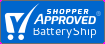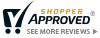Save 5% Extra Online. Use code: SAV1127MenuSave 5% Extra Online. Use code: SAV1127More Battery Answers

Is a 20V Max more Powerful than 18V?

On first pull of the trigger the 20V Max drops to 18V and you get the nominal rating of 18V. This is because batteries are made up of smaller 3.6V (4V max) lithium cells which are regulated for exact outputs and used in a wide range of different electronics. This means all 10.8V=12V Max, all 18V = 20V Max, 36V = 40V Max.

Voltage is a mathematical measurement: V= I x R; where V=Voltage, I=Current, R=Resistance. In every battery different voltages can be measured. Voltage is an electrical measure of energy potential. If you do not charge the battery you can measure the peak voltage or max voltage higher than the nominal voltage. But batteries operate at nominal voltage which is lower than peak voltage. So is there a difference? Yes in the sense that the voltage measurement occurs at two different points within the battery - before and after battery operation. But no there is no difference in the actual battery. So why do companies market products at 20V with the word MAX instead of the operating voltage or nominal voltage which is 18V? Well as a marketer I would not know the answer!!!!!